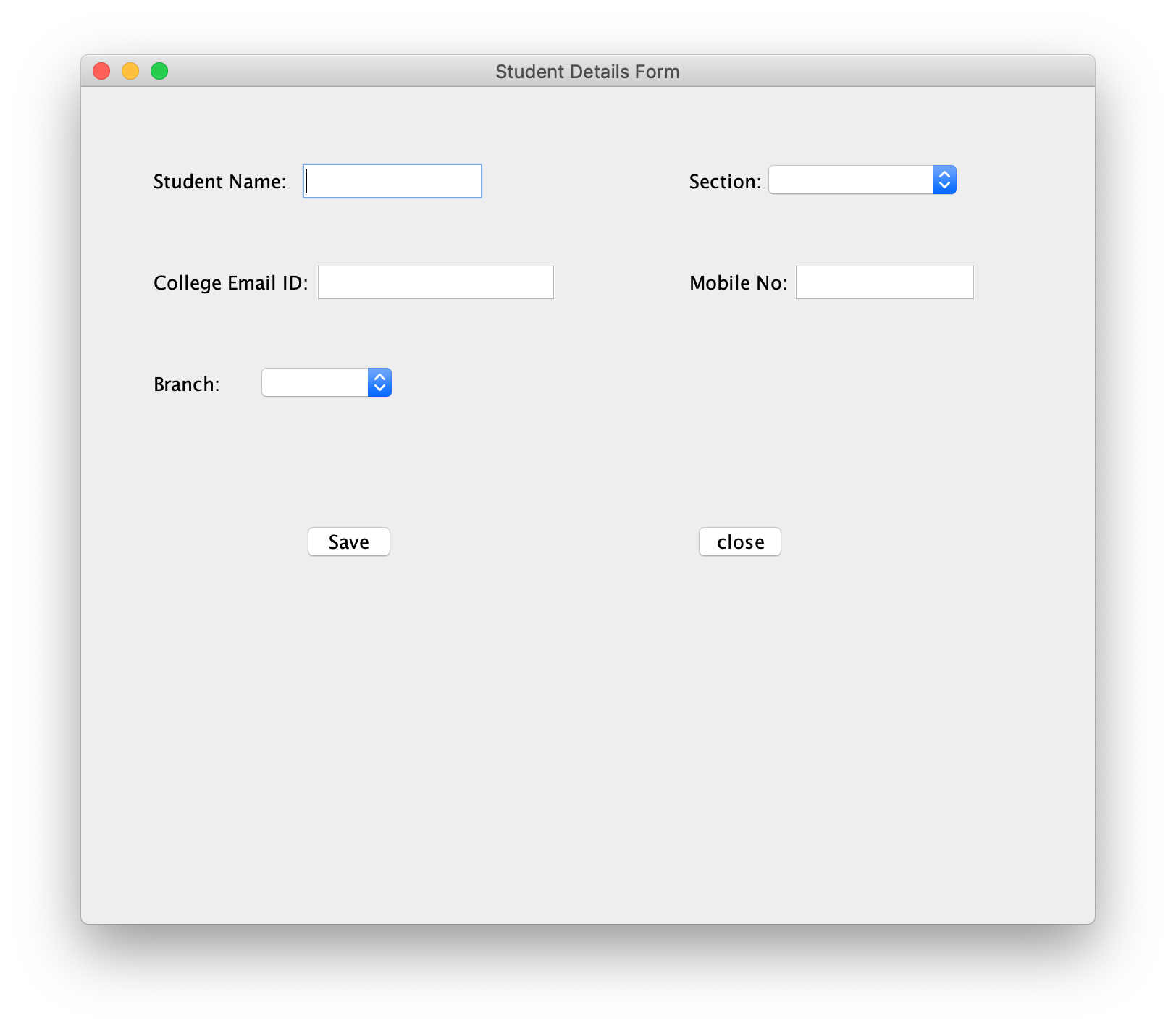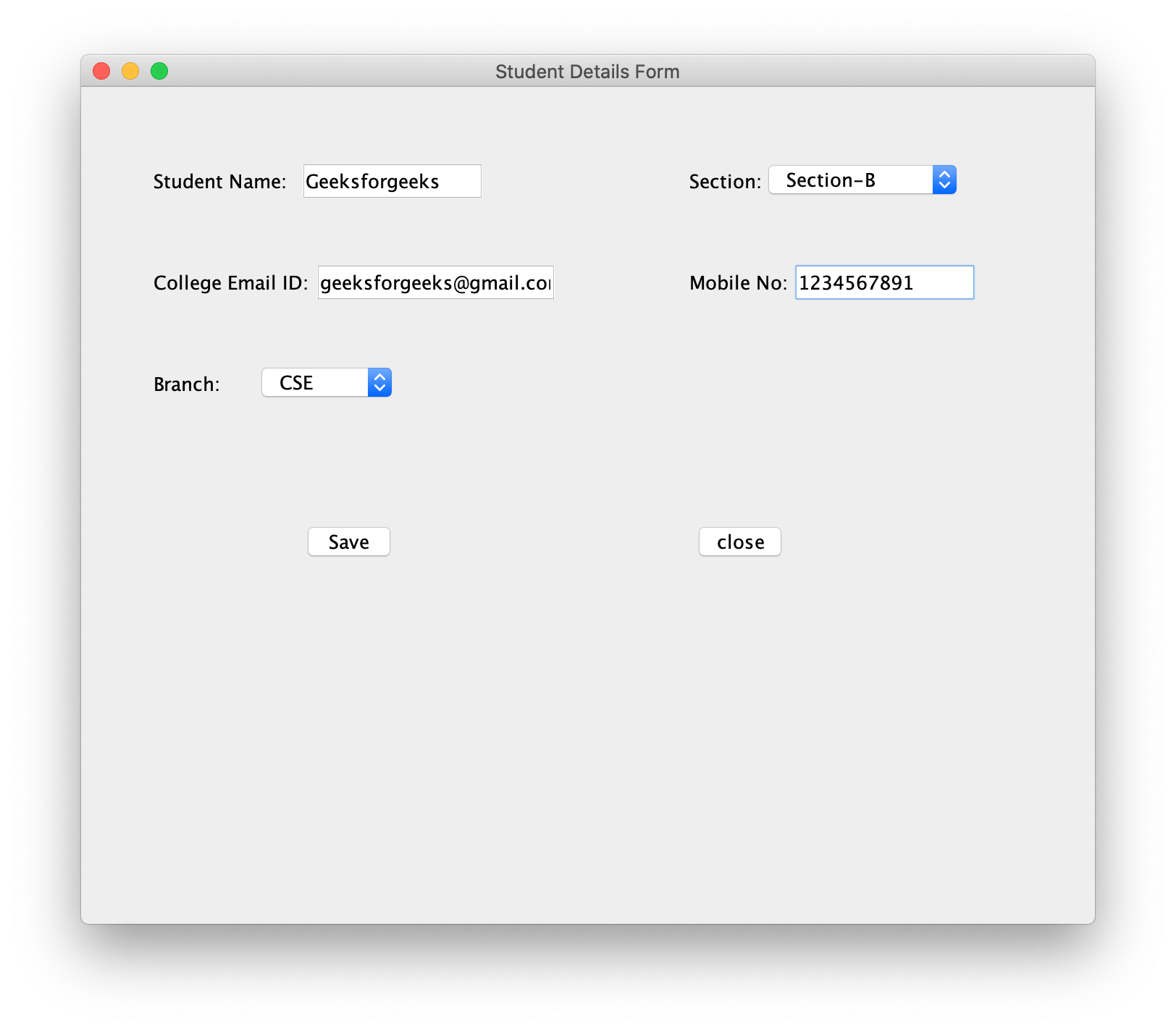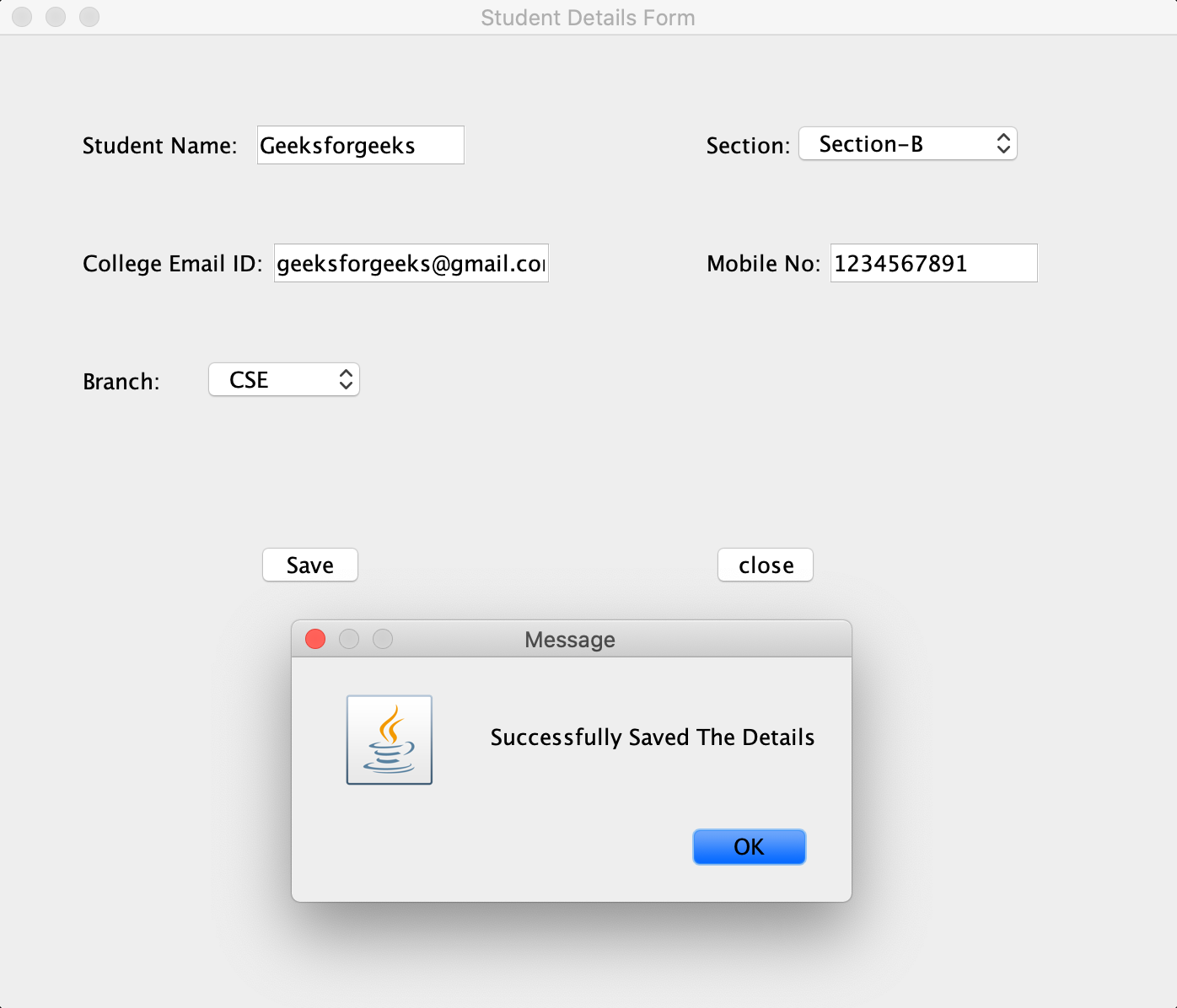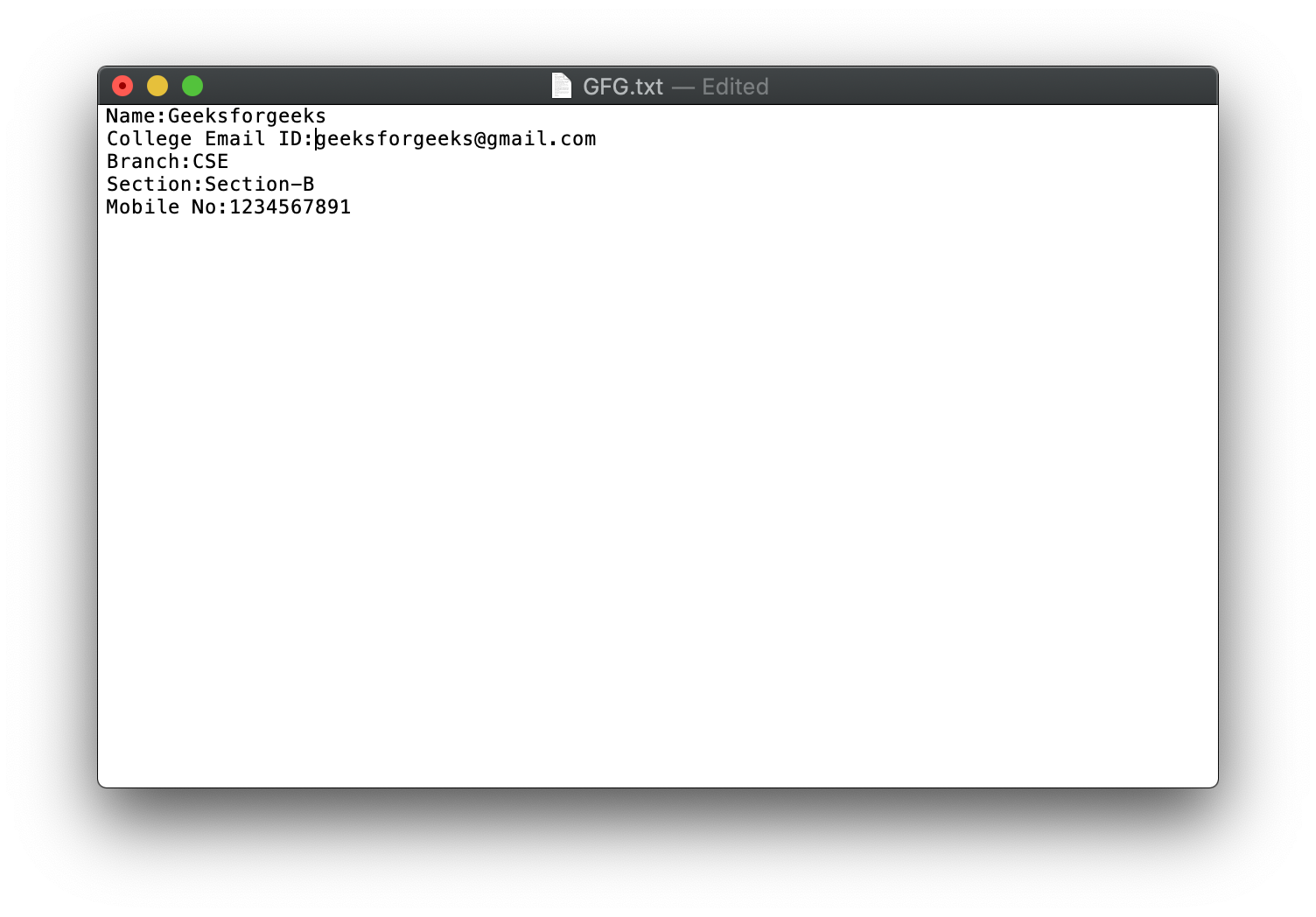Related Articles

# Java program to store a Student Information in a File using AWT

• Last Updated : 20 May, 2020

Swing is a part of the JFC (Java Foundation Classes). Building Graphical User Interface in Java requires the use of Swings. Swing Framework contains a large set of components which allow a high level of customization and provide rich functionalities, and is used to create window-based applications. Java swing components are lightweight, platform-independent, provide powerful components like tables, scroll panels, buttons, list, colour chooser, etc.

In this article, we will see how to write the students information in a Jframe and store it in a file.

## Recommended: Please try your approach on {IDE} first, before moving on to the solution.

Approach: To solve this problem, the following steps are followed:

1. First, we need to create a frame using JFrame.
2. Next create JLabels, JTextFields, JComboBoxes, JButtons and set their bounds respectively.
3. Name these components accordingly and set their bounds.
4. Now, in order to save the data into the text file on button click, we need to add Event Handlers. In this case, we will add ActionListener to perform an action method known as actionPerformed in which first we need to get the values from the text fields which is default as a “string”.
5. Finally, the Jbuttons, JLabels, JTextFields and JComboBoxes are added to the JFrame and the text is stored in a text file.

Below is the implementation of the above approach:

 `// Java program to write a student``// information in JFrame and``// storing it in a file`` ` `import` `javax.swing.*;``import` `java.awt.*;``import` `java.awt.event.*;``import` `java.io.*;`` ` `public` `class` `GFG {`` ` `    ``// Function to write a student``    ``// information in JFrame and``    ``// storing it in a file``    ``public` `static` `void` `StudentInfo()``    ``{`` ` `        ``// Creating a new frame using JFrame``        ``JFrame f``            ``= ``new` `JFrame(``                ``"Student Details Form"``);`` ` `        ``// Creating the labels``        ``JLabel l1, l2, l3, l4, l5;`` ` `        ``// Creating three text fields.``        ``// One for student name, one for``        ``// college mail ID  and one``        ``// for  Mobile No``        ``JTextField t1, t2, t3;`` ` `        ``// Creating two JComboboxes``        ``// one for Branch and one``        ``// for Section``        ``JComboBox j1, j2;`` ` `        ``// Creating  two buttons``        ``JButton b1, b2;`` ` `        ``// Naming the labels and setting``        ``// the bounds for the labels``        ``l1 = ``new` `JLabel(``"Student Name:"``);``        ``l1.setBounds(``50``, ``50``, ``100``, ``30``);``        ``l2 = ``new` `JLabel(``"College Email ID:"``);``        ``l2.setBounds(``50``, ``120``, ``120``, ``30``);``        ``l3 = ``new` `JLabel(``"Branch:"``);``        ``l3.setBounds(``50``, ``190``, ``50``, ``30``);``        ``l4 = ``new` `JLabel(``"Section:"``);``        ``l4.setBounds(``420``, ``50``, ``70``, ``30``);``        ``l5 = ``new` `JLabel(``"Mobile No:"``);``        ``l5.setBounds(``420``, ``120``, ``70``, ``30``);`` ` `        ``// Creating the textfields and``        ``// setting the bounds for textfields``        ``t1 = ``new` `JTextField();``        ``t1.setBounds(``150``, ``50``, ``130``, ``30``);``        ``t2 = ``new` `JTextField();``        ``t2.setBounds(``160``, ``120``, ``130``, ``30``);``        ``t3 = ``new` `JTextField();``        ``t3.setBounds(``490``, ``120``, ``130``, ``30``);`` ` `        ``// Creating two string arrays one for``        ``// braches and other for sections``        ``String s1[]``            ``= { ``"  "``, ``"CSE"``, ``"ECE"``, ``"EEE"``,``                ``"CIVIL"``, ``"MEC"``, ``"Others"` `};``        ``String s2[]``            ``= { ``"  "``, ``"Section-A"``, ``"Section-B"``,``                ``"Section-C"``, ``"Section-D"``,``                ``"Section-E"` `};`` ` `        ``// Creating two JComboBoxes one for``        ``// selecting branch and other for``        ``// selecting the section``        ``// and setting the bounds``        ``j1 = ``new` `JComboBox(s1);``        ``j1.setBounds(``120``, ``190``, ``100``, ``30``);``        ``j2 = ``new` `JComboBox(s2);``        ``j2.setBounds(``470``, ``50``, ``140``, ``30``);`` ` `        ``// Creating one button for Saving``        ``// and other button to close``        ``// and setting the bounds``        ``b1 = ``new` `JButton(``"Save"``);``        ``b1.setBounds(``150``, ``300``, ``70``, ``30``);``        ``b2 = ``new` `JButton(``"close"``);``        ``b2.setBounds(``420``, ``300``, ``70``, ``30``);`` ` `        ``// Adding action listener``        ``b1.addActionListener(``new` `ActionListener() {``            ``public` `void` `actionPerformed(ActionEvent e)``            ``{`` ` `                ``// Getting the text from text fields``                ``// and JComboboxes``                ``// and copying it to a strings`` ` `                ``String s1 = t1.getText();``                ``String s2 = t2.getText();``                ``String s3 = j1.getSelectedItem() + ``""``;``                ``String s4 = j2.getSelectedItem() + ``""``;``                ``String s5 = t3.getText();``                ``if` `(e.getSource() == b1) {``                    ``try` `{`` ` `                        ``// Creating a file and``                        ``// writing the data``                        ``// into a Textfile.``                        ``FileWriter w``                            ``= ``new` `FileWriter(``                                ``"GFG.txt"``, ``true``);`` ` `                        ``w.write(s1 + ``"\n"``);``                        ``w.write(s2 + ``"\n"``);``                        ``w.write(s3 + ``"\n"``);``                        ``w.write(s4 + ``"\n"``);``                        ``w.write(s5 + ``"\n"``);``                        ``w.close();``                    ``}``                    ``catch` `(Exception ae) {``                        ``System.out.println(ae);``                    ``}``                ``}`` ` `                ``// Shows a Pop up Message when``                ``// save button is clicked``                ``JOptionPane``                    ``.showMessageDialog(``                        ``f,``                        ``"Successfully Saved"``                            ``+ ``" The Details"``);``            ``}``        ``});`` ` `        ``// Action listener to close the form``        ``b2.addActionListener(``new` `ActionListener() {``            ``public` `void` `actionPerformed(ActionEvent e)``            ``{``                ``f.dispose();``            ``}``        ``});`` ` `        ``// Default method for closing the frame``        ``f.addWindowListener(``new` `WindowAdapter() {``            ``public` `void` `windowClosing(WindowEvent e)``            ``{``                ``System.exit(``0``);``            ``}``        ``});`` ` `        ``// Adding the created objects``        ``// to the frame``        ``f.add(l1);``        ``f.add(t1);``        ``f.add(l2);``        ``f.add(t2);``        ``f.add(l3);``        ``f.add(j1);``        ``f.add(l4);``        ``f.add(j2);``        ``f.add(l5);``        ``f.add(t3);``        ``f.add(b1);``        ``f.add(b2);``        ``f.setLayout(``null``);``        ``f.setSize(``700``, ``600``);``        ``f.setVisible(``true``);``    ``}``    ``// Driver code``    ``public` `static` `void` `main(String args[])``    ``{``        ``StudentInfo();``    ``}``}`

Output:

1. The window displayed on running the program:2. Entering the data:3. The dialog box showed after clicking on the save button:4. The text file in which the data is stored:Attention reader! Don’t stop learning now. Get hold of all the important Java Foundation and Collections concepts with the Fundamentals of Java and Java Collections Course at a student-friendly price and become industry ready. To complete your preparation from learning a language to DS Algo and many more,  please refer Complete Interview Preparation Course.

My Personal Notes arrow_drop_up